The Experiments of Faraday and Henry

Experiment 1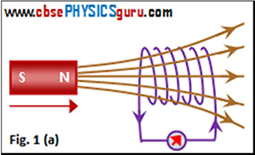When the North-pole of a bar magnet is moved towards the coil or loop, the galvanometer connected to the coil shows deflection. It confirms the flow of electric current in the coil. It is also noted that the deflection lasts as long as the bar magnet is in motion. No deflection is shown by the galvanometer when the magnet does not move. See figure 1(a). When the North-pole of the bar magnet is moved away from the coil, the galvanometer shows deflection in the opposite direction. It indicates that the current flows in the reverse direction. See figure 1(b).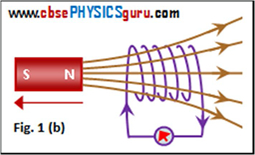If the same experiment is repeated with the South-pole of the bar magnet, the deflections shown by the galvanometer are just opposite to that observed with the North-pole for similar movements (towards the coil or away from the coil). In other words, if the South-pole is moved towards the coil, we will get the result shown in figure 1(b). If the South-pole is moved away from the coil, we will get the result shown in figure 1(a).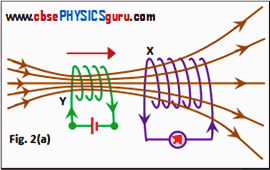The deflection, and consequently current becomes larger if the magnet is moved towards or away from the coil at a quicker rate.

Now, if the coil is moved towards or away from the stationary magnet, the same results are produced. It establishes that electric current in the coil is induced due to the relative motion between the magnet and the coil. It does not matter which is moving.

Experiment 2

If a second coil Y carrying a steady current drawn from a battery is moved towards the coil X connected to a galvanometer, then the galvanometer shows a deflection. This confirms that an electric 2 current is induced in coil X. See figure 2(a). The deflection in the galvanometer lasts as long as the coil Y is in motion.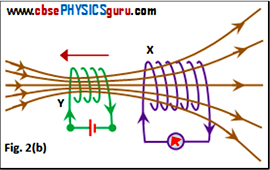When coil Y is moved away from the coil X, the galvanometer shows a deflection in the opposite direction. See figure 2(b). It is also noted that the deflection lasts as long as the coil Y is in motion. No deflection is shown by the galvanometer when the coil Y does not move.

Now, if the coil X is moved towards or away from the stationary coil Y, the same results are produced. It establishes that electric current in the coil X is induced due to the relative motion between the two coils. It does not matter which is moving.

In both the cases, the deflection, and consequently current becomes larger if a coil is moved towards or away from the other coil at a quicker rate.

Experiment 3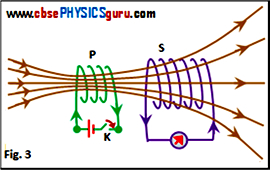Is relative motion between a magnet and a coil (experiment 1) and between two coils (experiment 2) is necessary to induce current? Faraday answered this question through another experiment and concluded that the relative motion is not an absolute requirement. As shown in figure 3, both the coils P and S are fixed. Coil S is connected to galvanometer while the coil P is connected to a battery through a key K. Coil P is generally called primary coil and coil S is called secondary coil. A momentary deflection is observed in the galvanometer when the key K is closed. The deflection shown by the galvanometer becomes zero almost instantly even if the key is kept closed to maintain current in the primary coil P.

Now, if the key is opened, a momentary deflection is again observed, but in the opposite direction. Clearly, the deflection the galvanometer is produced only when the key is closed or opened in the primary coil (or circuit or winding). It was concluded that the factor responsible for inducing current in the secondary coil and hence, the deflection in the galvanometer is the change in magnetic flux due to change in current in the primary coil.

It is also observed that the deflection increases when an iron rod is inserted into the coils along their axis.

CBSE Electromagnetic Induction ( With Hint / Solution)
Class XII (By Mr. Ashis Kumar Satapathy)
email - [email protected]

Electromagnetic Induction